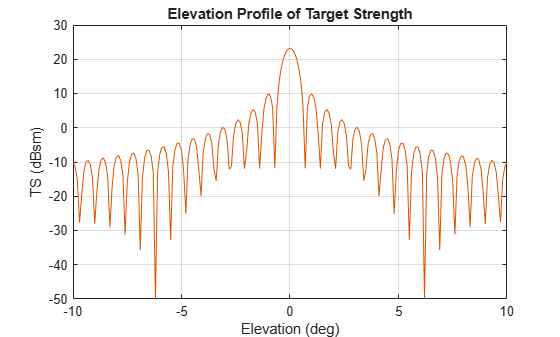Documentation

# tsSignature class

Target strength pattern

## Description

`tsSignature` creates a sonar target strength (TS) signature object. You can use this object to model an angle-dependent and frequency-dependent target strength pattern. Target strength determines the intensity of reflected sound signal power from a target.

## Construction

`tssig = tsSignature` creates a `tsSignature` object with default property values.

`tssig = tsSignature(Name,Value)` sets object properties using one or more `Name,Value` pair arguments. `Name` is a property name and `Value` is the corresponding value. `Name` must appear inside single quotes (`''`). You can specify several name-value pair arguments in any order as `Name1,Value1,...,NameN,ValueN`. Any unspecified properties take default values.

### Note

You can only set property values of `tsSignature` when constructing the object. The property values are not changeable after construction.

## Properties

expand all

Sampled target strength pattern, specified as a scalar, a Q-by-P real-valued matrix, or a Q-by-P-by-K real-valued array. The pattern is an array of TS values defined on a grid of elevation angles, azimuth angles, and frequencies. Azimuth and elevation are defined in the body frame of the target.

• Q is the number of TS samples in elevation.

• P is the number of TS samples in azimuth.

• K is the number of TS samples in frequency.

Q, P, and K usually match the length of the vectors defined in the `Elevation`, `Azimuth`, and `Frequency` properties, respectively, with these exceptions:

• To model a TS pattern for an elevation cut (constant azimuth), you can specify the TS pattern as a Q-by-1 vector or a 1-by-Q-by-K matrix. Then, the elevation vector specified in the `Elevation` property must have length 2.

• To model a TS pattern for an azimuth cut (constant elevation), you can specify the TS pattern as a 1-by-P vector or a 1-by-P-by-K matrix. Then, the azimuth vector specified in the `Azimuth` property must have length 2.

• To model a TS pattern for one frequency, you can specify the TS pattern as a Q-by-P matrix. Then, the frequency vector specified in the `Frequency` property must have length 2.

Example: `[10,0;0,-5]`

Data Types: `double`

Azimuth angles used to define the angular coordinates of each column of the matrix or array specified by the `Pattern` property. Specify the azimuth angles as a length-P vector. P must be greater than two. Angle units are in degrees.

Example: `[-45:0.1:45]`

Data Types: `double`

Elevation angles used to define the coordinates of each row of the matrix or array specified by the `Pattern` property. Specify the elevation angles as a length-Q vector. Q must be greater than two. Angle units are in degrees.

Example: `[-30:0.1:30]`

Data Types: `double`

Frequencies used to define the applicable RCS for each page of the `Pattern` property. Specify the frequencies as a length-K vector. K must be greater than two. Frequency units are in hertz.

Example: `[-30:0.1:30]`

Data Types: `double`

## Methods

 value Target strength at specified angle and frequency

## Examples

collapse all

Specify the target strength (TS) of a 5m long rigid cylinder immersed in water and plot TS values along an azimuth cut. Assume the short-wavelength approximation. The cylinder radius is 2m. The speed of sound is 1520 m/s.

```L = 5; a = 2;```

Create an array of target strengths at two wavelengths. First, specify the range of azimuth and elevation angles over which TS is defined. Then, use an analytical model to compute the target strength. Create an image of the TS.

```lambda = [0.12, .1]; c = 1520.0; az = [-20:0.1:20]; el = [-10:0.1:10]; ts1 = ts_cylinder(L,a,az,el,lambda(1)); ts2 = ts_cylinder(L,a,az,el,lambda(2)); tsdb1 = 10*log10(ts1); tsdb2 = 10*log10(ts2); imagesc(az,el,tsdb1) title('Target Strength') xlabel('Azimuth (deg)') ylabel('Elevation (deg)') colorbar```Create a `tsSignature` object and plot an elevation cut at $3{0}^{\circ }$ azimuth.

```tsdb(:,:,1) = tsdb1; tsdb(:,:,2) = tsdb2; freq = c./lambda; tssig = tsSignature('Pattern',tsdb,'Azimuth',az,'Elevation',el,'Frequency',freq); ts = value(tssig,30,el,freq(1)); plot(el,tsdb1) grid title('Elevation Profile of Target Strength') xlabel('Elevation (deg)') ylabel('TS (dBsm)')``````function ts = ts_cylinder(L,a,az,el,lambda) k = 2*pi/lambda; beta = k*L*sind(el')*ones(size(az)); gamma = cosd(el')*ones(size(az)); ts = a*L^2*(sinc(beta).^2).*gamma.^2/2/lambda; ts = max(ts,10^(-5)); end function s = sinc(theta) s = ones(size(theta)); idx = (abs(theta) <= 1e-2); s(idx) = 1 - 1/6*(theta(idx)).^2; s(~idx) = sin(theta(~idx))./theta(~idx); end```

## References

 Urich, Robert J. Principles of Underwater Sound, 3rd ed. New York: McGraw-Hill, Inc. 2005.